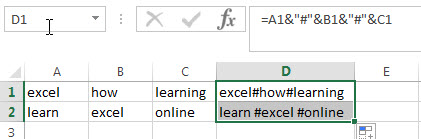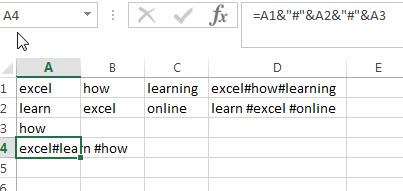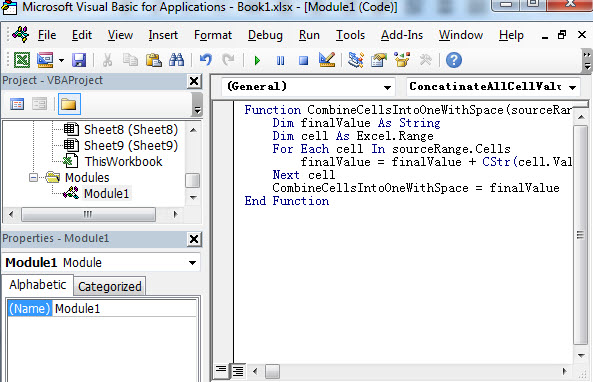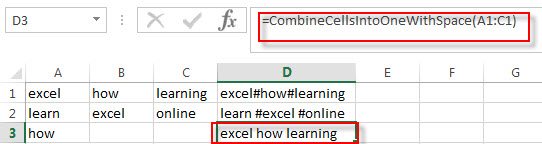# Combining multiple cells into one cell with a specified character

This post will guide you how to combine multiple cells in a row or column into one cell with a specified character, such as: comma, hash, space, semicolon and so on. How to achieve it? And this post will let you learn two ways to combine or merge range of cells in a row or column into one cell in excel.

## Combining multiple cells into one with hash character using excel formula

Assuming that you want to combine a range of cells within a row, such as: A1:A3. You can use the concatenation operator to create an excel formula to merge multiple cells in a row into one cell with a specified character hash sign.

You can enter the following formula into a blank cell, such as: D1 and then press Enter key to apply this formula.

`=A1&"#"&B1&"#"&C1`Of course, if you want to combine range of cells (A1:A3) in a column into one cell (A4), then you can try to enter the below formula into Cell A4, and then press Enter key to apply this formula.

`=A1&"#"&A2&"#"&A3`## Combining multiple cells into one with hash character using VBA User defined function

You can also write a User Defined Function in excel VBA to merge the range of cells in a row or column into one cell.

1# open your excel workbook and then click on “Visual Basic” command under DEVELOPER Tab, or just press “ALT+F11” shortcut.2# then the “Visual Basic Editor” window will appear.

3# click “Insert” ->”Module” to create a new module4# paste the below VBA code into the code window. Then clicking “Save” button.```Function CombineCellsIntoOneWithSpace(sourceRange As Excel.Range) As String
Dim finalValue As String
Dim cell As Excel.Range
For Each cell In sourceRange.Cells
finalValue = finalValue + CStr(cell.Value) + " "
Next cell
CombineCellsIntoOneWithSpace = finalValue
End Function```

5# back to the current worksheet, try to enter the below formula to merge the range of cells A1:C1 to only one cell D3.

`=CombineCellsIntoOneWithSpace(A1:A3)`### Related Posts

• Combine Text from Two or More Cells into One Cell
If you want to combine text from multiple cells into one cell and you can use the Ampersand (&) symbol. If you want to join the text from multiple cells into one cell, you also can use the CONCATENATE function instead of Ampersand operator. ..…
• Combine 3 Cells to Generate One Date
If you want to combine 3 different cells to generate a standard data, you can create an excel formula based on the DATE function..…
• How to combine columns without losing data
How to keep all data after merging columns. You can use the concatenate operator or CONCATENATE function to create an excel formula.…
• Merge multiple worksheets into one worksheet
How to merge two or more excel worksheet into one worksheet by using some VBA code. You can create a new excel macro to combine multiple worksheets into one worksheet in Excel VBA…
• Combine multiple workbooks into one workbook
If you want to combine multiple workbooks into one workbook, you need to open all workbooks, then determine the workbooks to merge and the destination of workbook.…